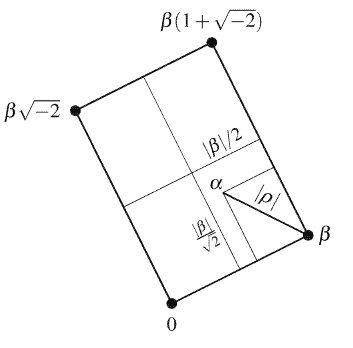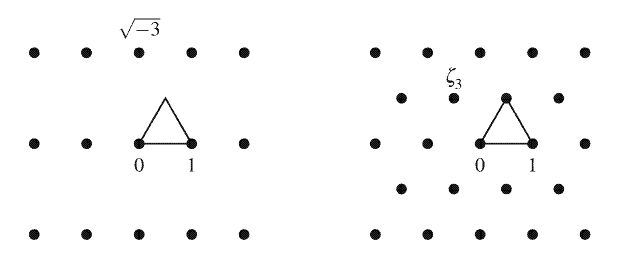## PREVIEW

Just as Gaussian integers enable the factorization of $x^{2}+y^{2}$, other quadratic expressions in ordinary integer variables are factorized with the help of quadratic integers. Examples in this chapter are
\begin{aligned} x^{2}+2 &=(x-\sqrt{-2})(x+\sqrt{-2}) \ x^{2}-x y+y^{2} &=\left(x+\frac{-1+\sqrt{-3}}{2} y\right)\left(x+\frac{-1-\sqrt{-3}}{2} y\right) \end{aligned}
In the first example, the factors belong to
$$\mathbb{Z}[\sqrt{-2}]={a+b \sqrt{-2}: a, b \in \mathbb{Z}}$$
Like the Gaussian integers $a+b i$, the numbers $a+b \sqrt{-2}$ enjoy unique prime factorization. We use this property to find all (ordinary) integer solutions of the equation $y^{3}=x^{2}+2$.
The numbers $\frac{-1+\sqrt{-3}}{2}$ and $\frac{-1-\sqrt{-3}}{2}$ in the second example appear at first to be “fractional”, and one might prefer to reserve the term “integer” for numbers in
$$\mathbb{Z}[\sqrt{-3}]={a+b \sqrt{-3}: a, b \in \mathbb{Z}}$$
However, unique prime factorization fails in $\mathbb{Z}[\sqrt{-3}]$, and it is precisely by adjoining the number $\frac{-1+\sqrt{-3}}{2}$ that it is regained.

This leads to a discussion (partly in the exercises) of the general concept of quadratic integer and its applications. The chapter concludes with two remarkable applications of $\sqrt{-3}$ : parametric formulas for the (infinitely many) rational solutions of $x^{3}+y^{3}=z^{3}+w^{3}$, and proof that there are no nonzero integer solutions of $x^{3}+y^{3}=z^{3}$

## The equation $y^{3}=x^{2}+2$

Diophantus was usually interested in rational solutions of equations, and the equation $y^{3}=x^{2}+2$ has infinitely many of these . But in his Arithmetica, Book VI, Problem 17, Diophantus mentioned that $y^{3}=x^{2}+2$ has the solution $x=5, y=3 .$ He evidently thought that this natural number solution was interesting. In 1657 Fermat added the claim that there is no other natural number solution of $y^{3}=x^{2}+2$
Fermat’s claim was proved by Euler (1770), assuming unique prime factorization in
$$\mathbb{Z}[\sqrt{-2}]={a+b \sqrt{-2}: a, b \in \mathbb{Z}}$$
Euler gave no the proof of the latter fact (which is similar to the proof for $\mathbb{Z}[i]$ given in Section $6.4)$, but leaving unique factorization aside for the moment, his argument goes as follows.

Supposing that $y^{3}=x^{2}+2$ for some $x, y \in \mathbb{Z}$, we have a factorization in $\mathbb{Z}[\sqrt{-2}]$
$$y^{3}=(x-\sqrt{-2})(x+\sqrt{-2})$$
Now we assume unique prime factorization in $\mathbb{Z}[\sqrt{-2}]$ and also that
$$\operatorname{gcd}(x-\sqrt{-2}, x+\sqrt{-2})=1$$
(another fact that we leave aside for the moment). By considering the prime factorizations of both sides of $(*)$, it then follows that the factors $x-\sqrt{-2}$ and $x+\sqrt{-2}$ are cubes in $\mathbb{Z}[\sqrt{-2}]$.
The latter statement means that
\begin{aligned} x-\sqrt{-2} &=(a+b \sqrt{-2})^{3} \quad \text { for some } a, b \in \mathbb{Z} \ &=a^{3}+3 a^{2} b \sqrt{-2}-6 a b^{2}-2 \sqrt{-2} b^{3} \ &=a^{3}-6 a b^{2}+\left(3 a^{2} b-2 b^{3}\right) \sqrt{-2} \end{aligned}
Equating real and imaginary parts gives
\begin{aligned} &x=a^{3}-6 a b^{2} \ &1=2 b^{3}-3 a^{2} b=b\left(2 b^{2}-3 a^{2}\right) \end{aligned}
The latter equation says that the natural number $b$ divides 1, hence $b=\pm 1$. Then the other divisor $2 b^{2}-3 a^{2}$ of 1 must equal $-1$, hence $a=\pm b=\pm 1$.
This gives $x=\pm 5$ and hence $y=3$.

## The division property in $\mathbb{Z}[\sqrt{-2}]$

Unique prime factorization follows the same way as in $\mathbb{Z}$ and $\mathbb{Z}[i]:$ from the prime divisor property that follows from a Euclidean algorithm, made possible by a division property.
Division property for $\mathbb{Z}[\sqrt{-2}]$. For any $\alpha, \beta \neq 0$ in $\mathbb{Z}[\sqrt{-2}]$ there are $\mu$, $\rho$ in $\mathbb{Z}[\sqrt{-2}]$ with
$$\alpha=\mu \beta+\rho \quad \text { and } \quad|\rho|<|\beta|$$
Proof. To see why the division property holds in $\mathbb{Z}[\sqrt{-2}]$ we look at the multiples $\mu \beta$ of any nonzero $\beta \in \mathbb{Z}[\sqrt{-2}]$. These lie at the corners of a grid of rectangles, the first of which has corners at $0, \beta, \beta \sqrt{-2}$ and $\beta(1+\sqrt{-2})$, shown in Figure $7.1$

Any $\alpha \in \mathbb{Z}[\sqrt{-2}]$ is in one of these rectangles and, as the picture shows, the distance $|\rho|$ of $\alpha$ from the nearest multiple $\mu \beta$ of $\beta$ satisfies
\begin{aligned} |\rho|^{2} & \leq\left(\frac{|\beta|}{2}\right)^{2}+\left(\frac{|\beta|}{\sqrt{2}}\right)^{2} \text { by Pythagoras’ theorem } \ &=\frac{|\beta|^{2}+2|\beta|^{2}}{4}=\frac{3|\beta|^{2}}{4} \end{aligned}

Hence $|\rho|<|\beta|$, as required.
The units in $\mathbb{Z}[\sqrt{-2}]$, as in $\mathbb{Z}$, are just $\pm 1$. We prove this using the norm $a^{2}+2 b^{2}$ of $a+b \sqrt{-2}$. Units are elements of norm 1 , and $a^{2}+2 b^{2}=1$ only if $b=0$ and $a=\pm 1$.

Now suppose we have a factorization of a cube into relatively prime factors $s$ and $t$ in $\mathbb{Z}[\sqrt{-2}]:$
$$y^{3}=s t$$
Since $s$ and $t$ have no prime factor in common, the cubed prime factors of $y^{3}$ must separate into cubes inside $s$ and cubes inside $t$. There could also be unit factors in $s$ and $t$, but these can only be 1 or $-1$, both of which are cubes. Hence the relatively prime factors of a cube are themselves cubes.
This fills another gap in Euler’s solution of $y^{3}=x^{2}+2$. The only gap that now remains is to show that $\operatorname{gcd}(x-\sqrt{-2}, x+\sqrt{-2})=1$.The division property in $\mathbb{Z}[\sqrt{-2} \mid$

Hence $|\rho|<|\beta|$, as required.
The units in $\mathbb{Z}[\sqrt{-2}]$, as in $\mathbb{Z}$, are just $\pm 1$. We prove this using the norm $a^{2}+2 b^{2}$ of $a+b \sqrt{-2}$. Units are elements of norm 1 , and $a^{2}+2 b^{2}=1$ only if $b=0$ and $a=\pm 1$.

Now suppose we have a factorization of a cube into relatively prime factors $s$ and $t$ in $\mathbb{Z}[\sqrt{-2}]$ :
$$y^{3}=s t$$
Since $s$ and $t$ have no prime factor in common, the cubed prime factors of $y^{3}$ must separate into cubes inside $s$ and cubes inside $t$. There could also be unit factors in $s$ and $t$, but these can only be 1 or $-1$, both of which are cubes. Hence the relatively prime factors of a cube are themselves cubes.
This fills another gap in Euler’s solution of $y^{3}=x^{2}+2$. The only gap that now remains is to show that $\operatorname{gcd}(x-\sqrt{-2}, x+\sqrt{-2})=1$

## $\mathbb{Z}[\sqrt{-3}]$ and $\mathbb{Z}\left[\zeta_{3}\right]$

A natural step after investigating $\mathbb{Z}[i]$ and $\mathbb{Z}[\sqrt{-2}]$ would be to study
$$\mathbb{Z}[\sqrt{-3}]={a+b \sqrt{-3}: a, b \in \mathbb{Z}}$$
But here is a surprise: unique prime factorization fails in $\mathbb{Z}[\sqrt{-3}]$ Consider the following factorizations of $4:$
$$4=2 \times 2=(1-\sqrt{-3})(1+\sqrt{-3})$$
In $\mathbb{Z}[\sqrt{-3}]$ the norm is
$$\operatorname{norm}(a+b \sqrt{-3})=|a+b \sqrt{-3}|^{2}=a^{2}+3 b^{2}$$
and, as usual, if $\alpha$ divides $\gamma$ then $\operatorname{norm}(\alpha)$ divides $\operatorname{norm}(\gamma)$.
But now $\operatorname{norm}(2)=4$, which is not divisible by any smaller integer of the form $a^{2}+3 b^{2}$ except 1 , hence 2 is a prime of $\mathbb{Z}[\sqrt{-2}]$. And
$$\operatorname{norm}(1-\sqrt{-3})=1+3=4$$
so $1-\sqrt{-3}$ is also prime, as is $1+\sqrt{-3}$. Thus 4 has two distinct prime factorizations in $\mathbb{Z}[\sqrt{-3}]$
This defect can be repaired by enlarging $\mathbb{Z}[\sqrt{-3}]$ to
$$\mathbb{Z}\left[\zeta_{3}\right]=\left{a+b \zeta_{3}: a, b \in \mathbb{Z}\right}$$
where
$$\zeta_{3}=\frac{-1+\sqrt{-3}}{2}$$
is one of the imaginary cube roots of 1 . (This is why we use the subscript 3. In general, $\zeta_{n}$ denotes $\cos \frac{2 \pi}{n}+i \sin \frac{2 \pi}{n}$, the $n$th root of 1.) The elements of $\mathbb{Z}\left[\zeta_{3}\right]$ lie at the corners of a tiling of the plane by equilateral triangles, and are called the Eisenstein integers.

By geometric arguments like those used for $\mathbb{Z}[i]$ and $\mathbb{Z}[\sqrt{-2}]$, we can see that $\mathbb{Z}\left[\zeta_{3}\right]$ has the division property, hence a Euclidean algorithm and unique prime factorization. Figure $7.2$ compares the point sets $\mathbb{Z}[\sqrt{-3}]$ and $\mathbb{Z}\left[\zeta_{3}\right]$ in the plane, showing why the division property fails for the first and succeeds for the second.

In the rectangles of $\mathbb{Z}[\sqrt{-3}]$, each center point (such as the one at the top of the triangle shown) is at distance 1 from the two nearest corners,$\mathbb{Z}[\sqrt{-3}]$ (left) and $\mathbb{Z}\left[\zeta_{3}\right]($ right $)$

hence its distance from the nearest corner is not less than the smallest side length of the rectangle. $\mathbb{Z}\left[\zeta_{3}\right]$ fills these “holes” in $\mathbb{Z}[\sqrt{-3}]$, producing the tiling of the plane by equilateral triangles.

Division property for $\mathbb{Z}\left[\zeta_{3}\right] .$ For any $\alpha, \beta \neq 0$ in $\mathbb{Z}\left[\zeta_{3}\right]$ there are $\mu, \rho$ in $\mathbb{Z}\left[\zeta_{3}\right]$ with
$$\alpha=\mu \beta+\rho \quad \text { and } \quad|\rho|<|\beta|$$
Proof. In the equilateral tiling, each point of the plane lies in some triangle and its distance from the nearest vertex is less than the side length of the triangle. In fact, its distance from any vertex of the surrounding triangle is less than the side length. This is so because a circle centered on a vertex and with a side as radius encloses the whole triangle.

This geometric property is the essence of the division property because, as usual, the set $\mu \beta$ of multiples of any nonzero $\beta \in \mathbb{Z}\left[\zeta_{3}\right]$ is the same shape as $\mathbb{Z}\left[\zeta_{3}\right]$. Its points are at the vertices of a tiling by equilateral triangles of side length $|\beta|$. Hence the distance $|\rho|=|\alpha-\mu \beta|$ from any $\alpha \in \mathbb{Z}\left[\zeta_{3}\right]$ to the nearest $\mu \beta$ is less than the side length $|\beta|$

There are six units in $\mathbb{Z}\left[\zeta_{3}\right]: \pm 1, \pm \zeta_{3}$ and $\pm \zeta_{3}^{2}$, and they lie at vertices of a hexagon on the unit circle with center at $O$ (see Figure $7.2$ again). Like the units in $\mathbb{Z}$ and $\mathbb{Z}[i]$, they all divide 1 . The two distinct factorizations of 4 in $\mathbb{Z}[\sqrt{-3}]$ are the “same” in $\mathbb{Z}\left[\zeta_{3}\right]$, up to unit factors, thanks to the extra units in the latter.

## Rational solutions of $x^{3}+y^{3}=z^{3}+w^{3}$

Like the Pythagorean equation $x^{2}+y^{2}=z^{2}$, the equation $x^{3}+y^{3}=z^{3}+w^{3}$ has infinitely many integer solutions, some of them of great renown. Here is one, associated with the great Indian number theorist Ramanujan.
It was Littlewood who said that every positive integer was one of Ramanujan’s personal friends. I remember going to see him once when he was lying ill at Putney. I had ridden in taxi-cab number 1729 , and remarked that the number seemed to me rather a dull one, and that I hoped it was not an unfavorable omen. “No,” he replied, “it is a very interesting number; it is the smallest number expressible as the sum of two cubes in two different ways.”

Hardy (1937).
The “two different ways” referred to by Ramanujan are
$$1729=9^{3}+10^{3}=1^{3}+12^{3}$$

thus they correspond to an integer solution of $x^{3}+y^{3}=z^{3}+w^{3}$. According to Brouncker (1657), the same solution was found by Frenicle, along with
$$\begin{array}{r} 9^{3}+15^{3}=2^{3}+16^{3} \ 15^{3}+33^{3}=2^{3}+34^{3} \ 16^{3}+33^{3}=9^{3}+34^{3} \ 19^{3}+24^{3}=10^{3}+27^{3} \end{array}$$
Another startling solution of this equation is $x=3, y=4, z=-5, w=6$ in other words $3^{3}+4^{3}+5^{3}=6^{3}$. This result, which seems to “generalize” $3^{2}+4^{2}=5^{2}$, really belongs to the equation $x^{3}+y^{3}=z^{3}+w^{3}$, but the latter resembles the Pythagorean equation in one respect-there is a parametric formula for all its rational solutions.

The formula is due to Euler (1756). His method can be simplified using complex numbers, namely the norm in $\mathbb{Q}[\sqrt{-3}]={a+b \sqrt{-3}: a, b \in \mathbb{Q}}$.
Parametric solution of $x^{3}+y^{3}=z^{3}+w^{3} .$ The rational solutions are
\begin{aligned} &x=\left[(p+3 q)\left(p^{2}+3 q^{2}\right)-1\right] r \ &y=\left[(-p+3 q)\left(p^{2}+3 q^{2}\right)+1\right] r \ &z=\left[-p+3 q+\left(p^{2}+3 q^{2}\right)^{2}\right] r \ &w=\left[p+3 q-\left(p^{2}+3 q^{2}\right)^{2}\right] r \end{aligned}
where $p, q$ and $r$ run through all rational numbers.
Proof. If we make the substitutions $x=X+Y, y=X-Y, z=Z+W$, $w=Z-W$, then the equation $x^{3}+y^{3}=z^{3}+w^{3}$ becomes
$$X\left(X^{2}+3 Y^{2}\right)=Z\left(Z^{2}+3 W^{2}\right)$$
and $X, Y, Z, W$ are rational if and only if $x, y, z, w$ are.
Thus the problem is equivalent to finding the rational solutions of the equation $X\left(X^{2}+3 Y^{2}\right)=Z\left(Z^{2}+3 W^{2}\right) .$ Also, we can specialize to $Z=1$ (if we later multiply the solution by an arbitrary rational), so it suffices to find the rational solutions of
$$X=\frac{1+3 W^{2}}{X^{2}+3 Y^{2}}$$

Now $a^{2}+3 b^{2}=|a+b \sqrt{-3}|^{2}$. Hence
\begin{aligned} X &=\frac{|1+W \sqrt{-3}|^{2}}{|X+Y \sqrt{-3}|^{2}} \ &=\left|\frac{1+W \sqrt{-3}}{X+Y \sqrt{-3}}\right|^{2} \quad \text { since the norm is multiplicative } \ &=|p+q \sqrt{-3}|^{2}=p^{2}+3 q^{2} \end{aligned}
for some $p, q$ that are rational if $X, Y, W$ are.
We can define $p$ and $q$ as the real and imaginary parts of
$$p+q \sqrt{-3}=\frac{1+W \sqrt{-3}}{X+Y \sqrt{-3}}$$
Multiplying both sides by $X+Y \sqrt{-3}$ gives
$$p X-3 q Y+(q X+p Y) \sqrt{-3}=1+W \sqrt{-3}$$
and therefore, equating real and imaginary parts,
$$p X-3 q Y=1, \quad q X+p Y=W$$
Since these are linear equations in $Y$ and $W$, we can solve for $Y$ and $W$ rationally in terms of $p, q$, and $X=p^{2}+3 q^{2}$. This gives a 1-to-1 correspondence between rational pairs $(p, q)$ and rational triples $(X, Y, W)$ such that $X\left(X^{2}+3 Y^{2}\right)=1+3 W^{2}$

Substituting these values of $X, Y, Z=1$, and $W$ back in $x, y, z, w$ we find that the rational solutions of $x^{3}+y^{3}=z^{3}+w^{3}$ are all the rational multiples of
\begin{aligned} &x=(p+3 q)\left(p^{2}+3 q^{2}\right)-1 \ &y=(-p+3 q)\left(p^{2}+3 q^{2}\right)+1 \ &z=-p+3 q+\left(p^{2}+3 q^{2}\right)^{2} \ &w=p+3 q-\left(p^{2}+3 q^{2}\right)^{2} \end{aligned}
as claimed.